####作者 | 真达、Mika

【导读】

Show me data，用数据说话。

1. 钱没给到位

2. 心受委屈了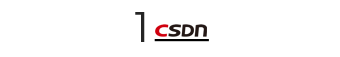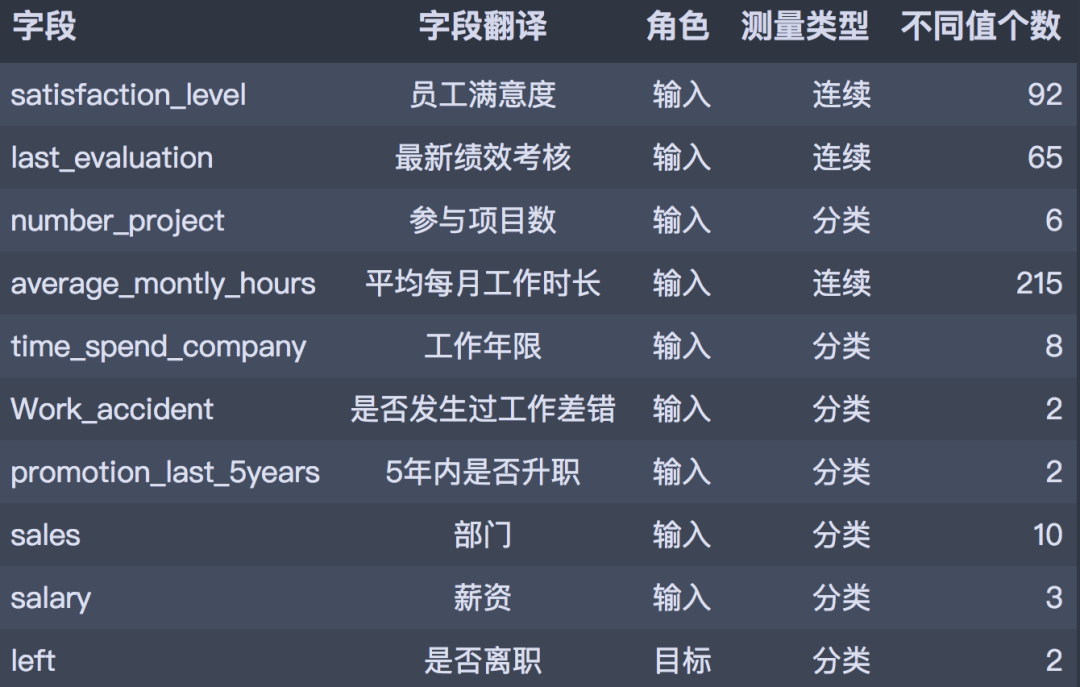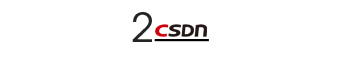``````# 导入包
import numpy as np
import pandas as pd
import matplotlib.pyplot as plt
import seaborn as sns

from pyecharts.charts import Bar, Pie, Page
from pyecharts import options as opts
from pyecharts.globals import SymbolType, WarningType
WarningType.ShowWarning = False
``````
``````plt.rcParams['font.sans-serif'] = ['SimHei']  # 用来正常显示中文标签
plt.rcParams['axes.unicode_minus'] = False  # 用来正常显示负号
``````
``````# 读入数据
``````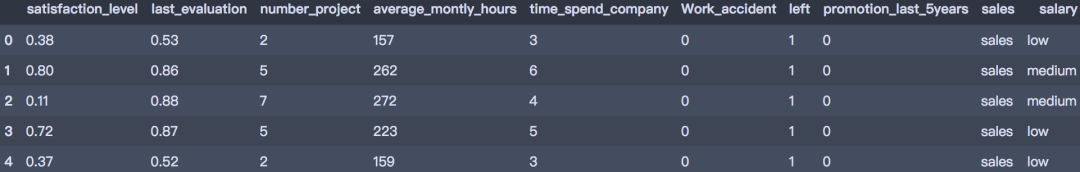``````df.info()
``````
``````<class 'pandas.core.frame.DataFrame'>
RangeIndex: 14999 entries, 0 to 14998
Data columns (total 10 columns):
#   Column                 Non-Null Count  Dtype
---  ------                 --------------  -----
0   satisfaction_level     14999 non-null  float64
1   last_evaluation        14999 non-null  float64
2   number_project         14999 non-null  int64
3   average_montly_hours   14999 non-null  int64
4   time_spend_company     14999 non-null  int64
5   Work_accident          14999 non-null  int64
6   left                   14999 non-null  int64
7   promotion_last_5years  14999 non-null  int64
8   sales                  14999 non-null  object
9   salary                 14999 non-null  object
dtypes: float64(2), int64(6), object(2)
memory usage: 1.1+ MB

``````
``````# 查看缺失值
print(df.isnull().any().sum())

``````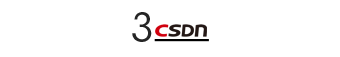1、 描述性统计

``````df.describe().T
``````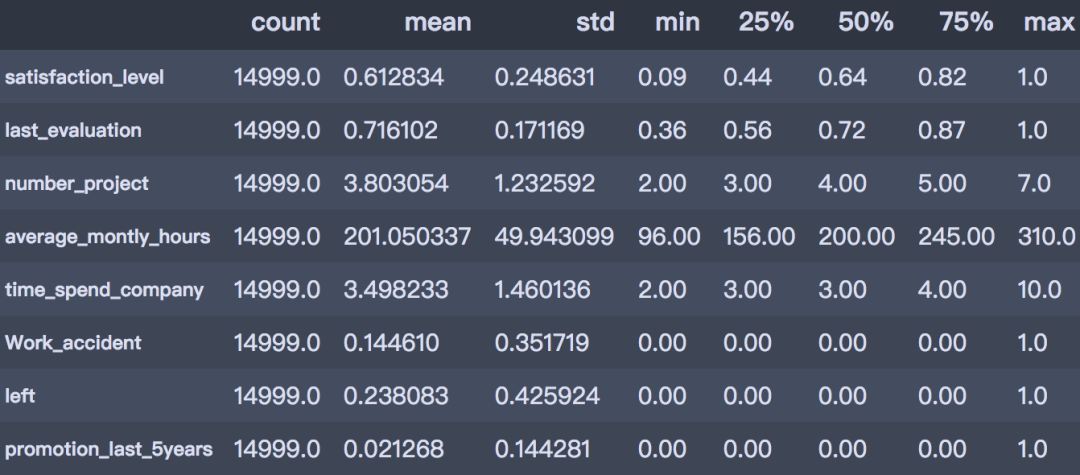• 员工满意度：范围 0.09~1, 中位数 0.640, 均值 0.613, 总体来说员工对公司比较满意；

• 绩效考核：范围 0.36~1, 中位数 0.72, 均值 0.716, 员工平均考核水平在中等偏上；

• 参与项目数：范围 2~7, 中位数 4, 均值 3.8, 平均参加项目数约 4 个；

• 平均每月工作时长：范围 96~310 小时, 中位数 200, 均值 201。

• 工作年限：范围 2~10 年, 中位数 3, 均值 3.5。

2、离职人数占比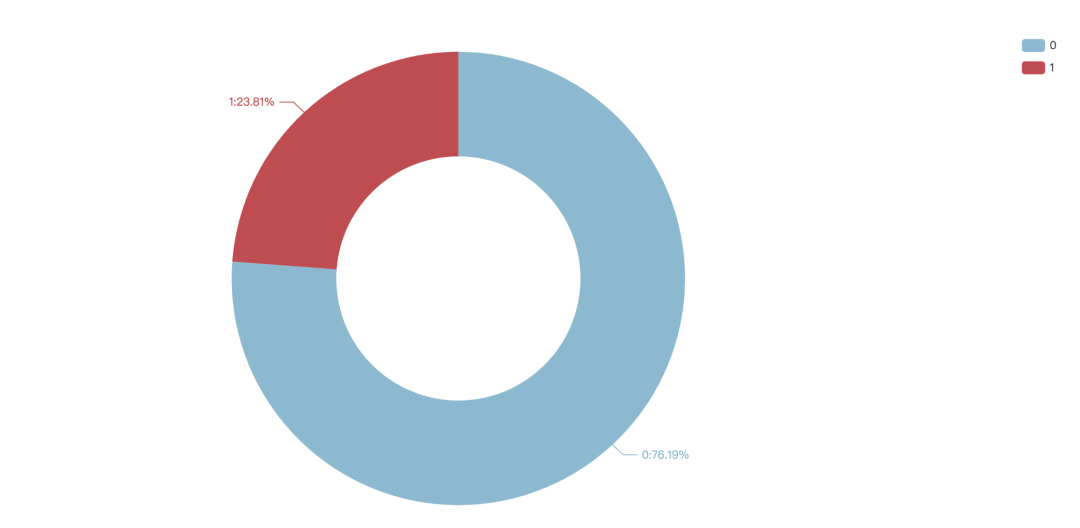3、员工满意度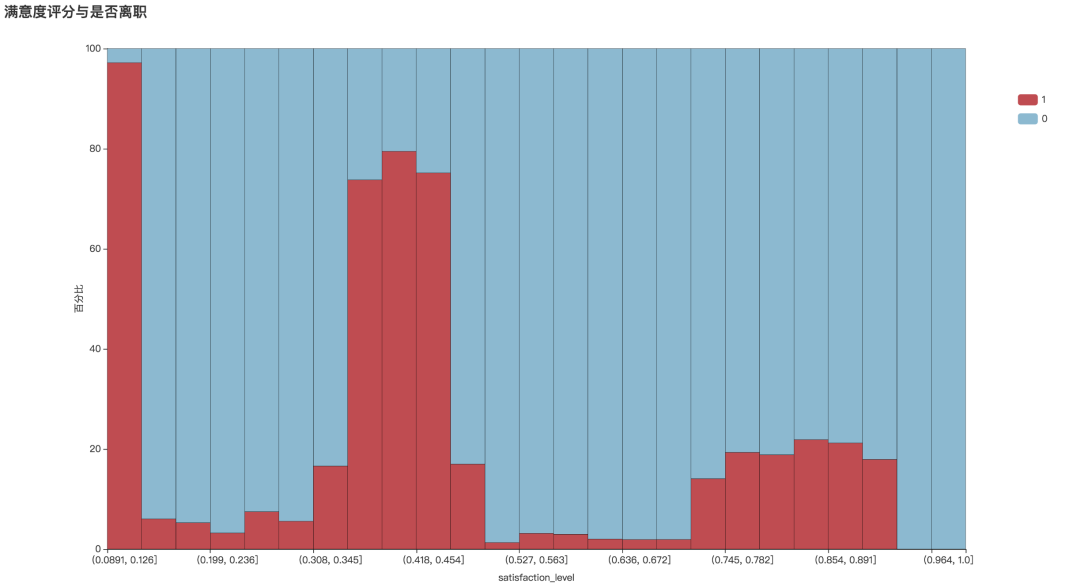``````df.groupby('left')['satisfaction_level'].describe()
``````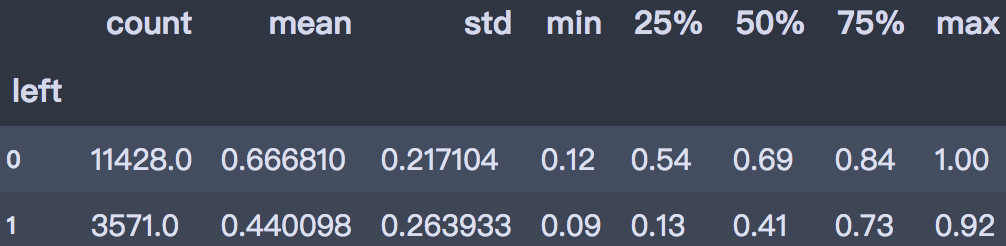``````def draw_numeric_graph(x_series, y_series, title):
# 产生数据
sat_cut = pd.cut(x_series, bins=25)
cross_table = round(pd.crosstab(sat_cut, y_series, normalize='index'),4)*100
x_data = cross_table.index.astype('str').tolist()
y_data1 = cross_table[cross_table.columns].values.tolist()
y_data2 = cross_table[cross_table.columns].values.tolist()

# 条形图
bar = Bar(init_opts=opts.InitOpts(width='1350px', height='750px'))
bar.set_global_opts(title_opts=opts.TitleOpts(title),
xaxis_opts=opts.AxisOpts(name=x_series.name, name_location='middle', name_gap=30),
yaxis_opts=opts.AxisOpts(name='百分比', name_location='middle', name_gap=30, min_=0, max_=100),
legend_opts=opts.LegendOpts(orient='vertical', pos_top='15%', pos_right='2%'))
bar.set_series_opts(label_opts=opts.LabelOpts(is_show=False),
itemstyle_opts=opts.ItemStyleOpts(border_color='black', border_width=0.3))
bar.set_colors(['#BF4C51', '#8CB9D0'])

return bar

``````
``````bar1 = draw_numeric_graph(df['satisfaction_level'], df['left'], title='满意度评分与是否离职')
bar1.render()

``````

4、绩效考核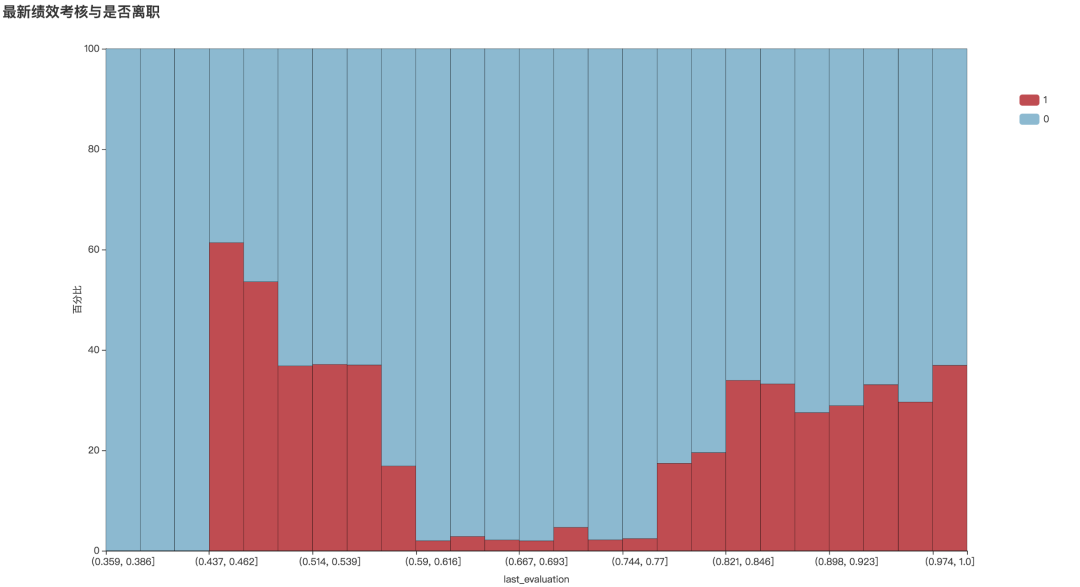5、平均每月工作时长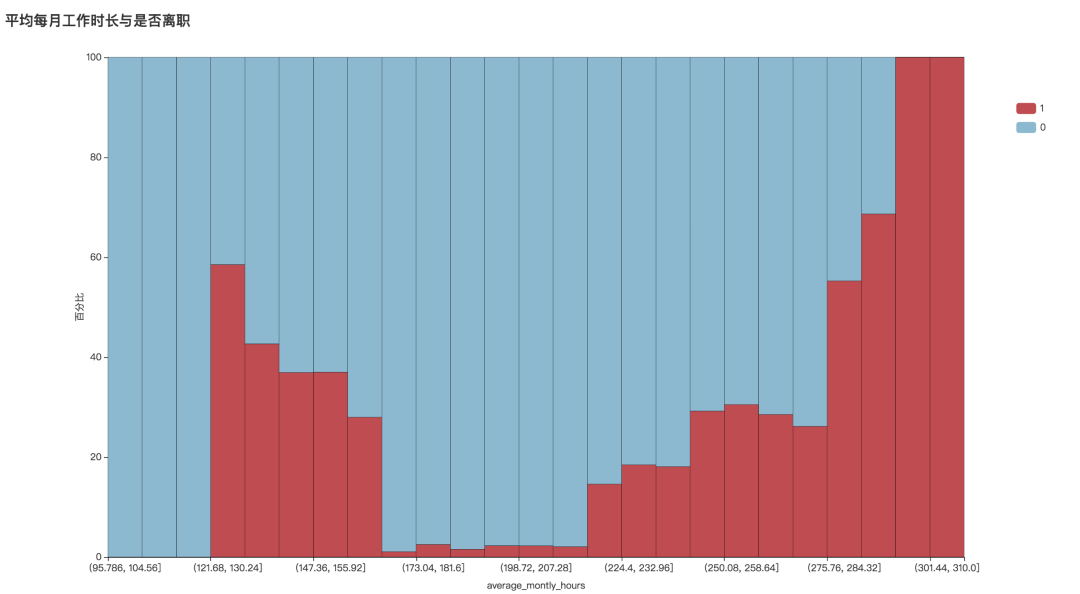6、参加项目数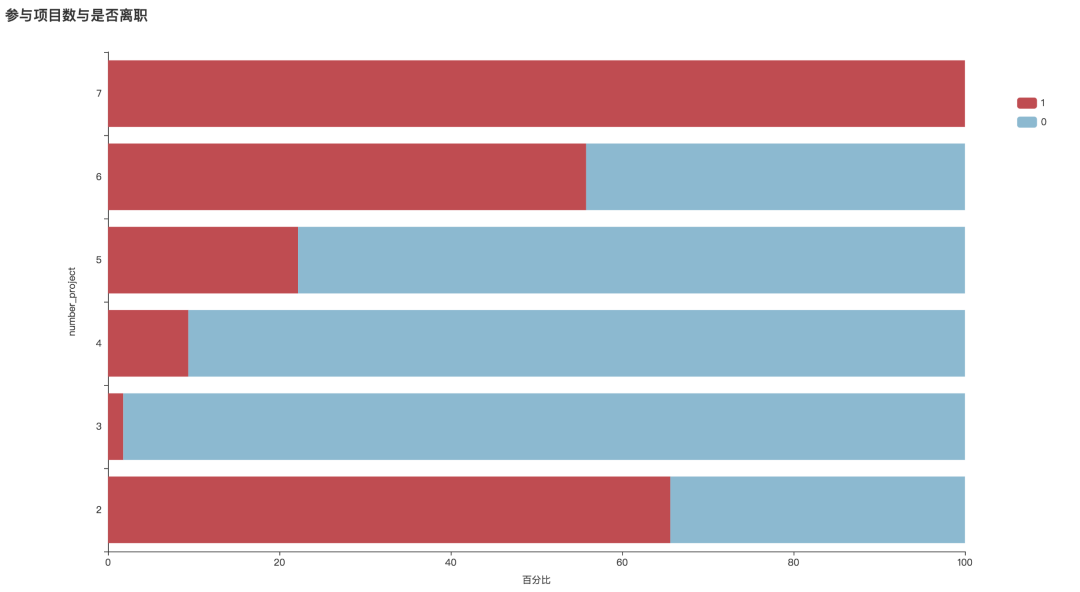7、员工工龄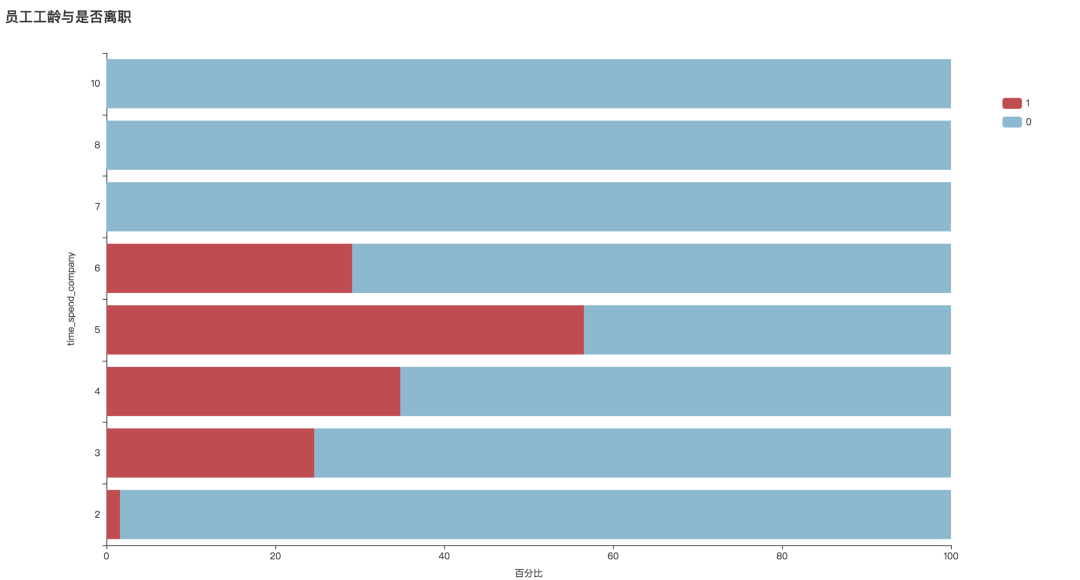8、工作事故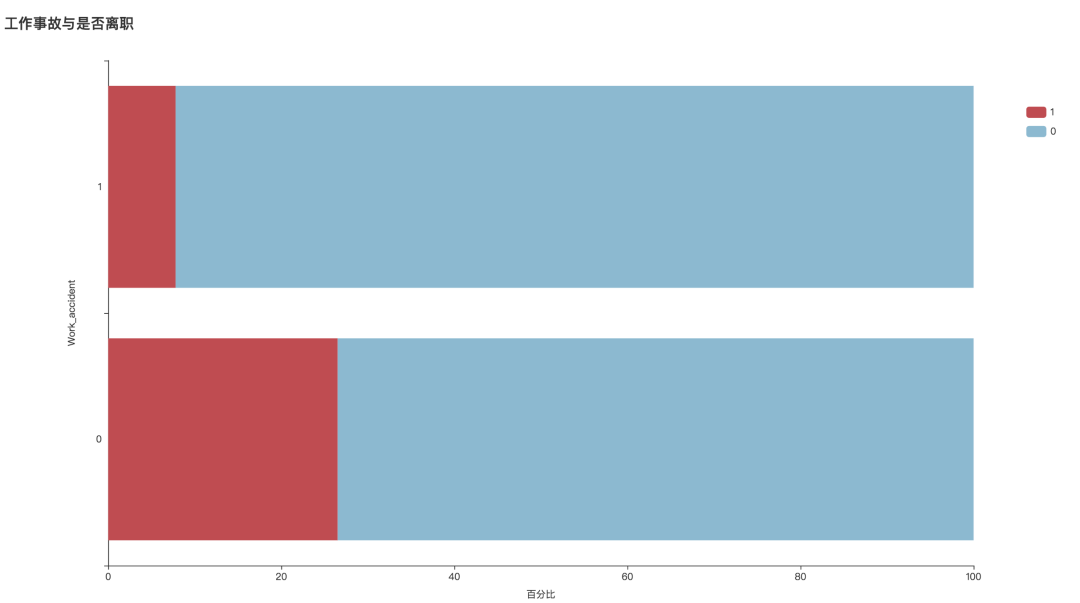9、职位晋升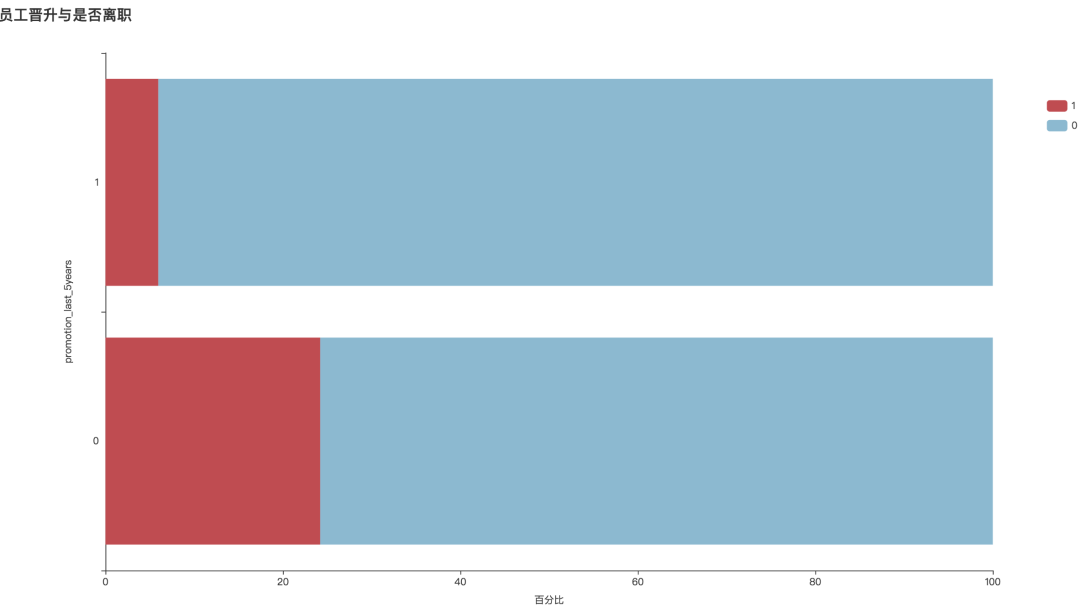10、薪资水平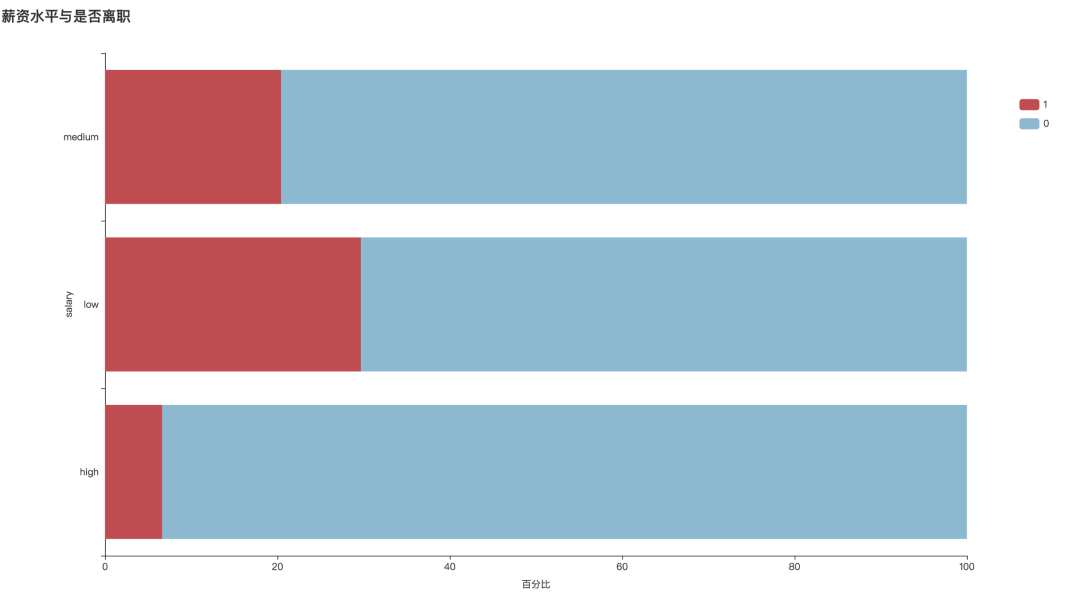11、不同部门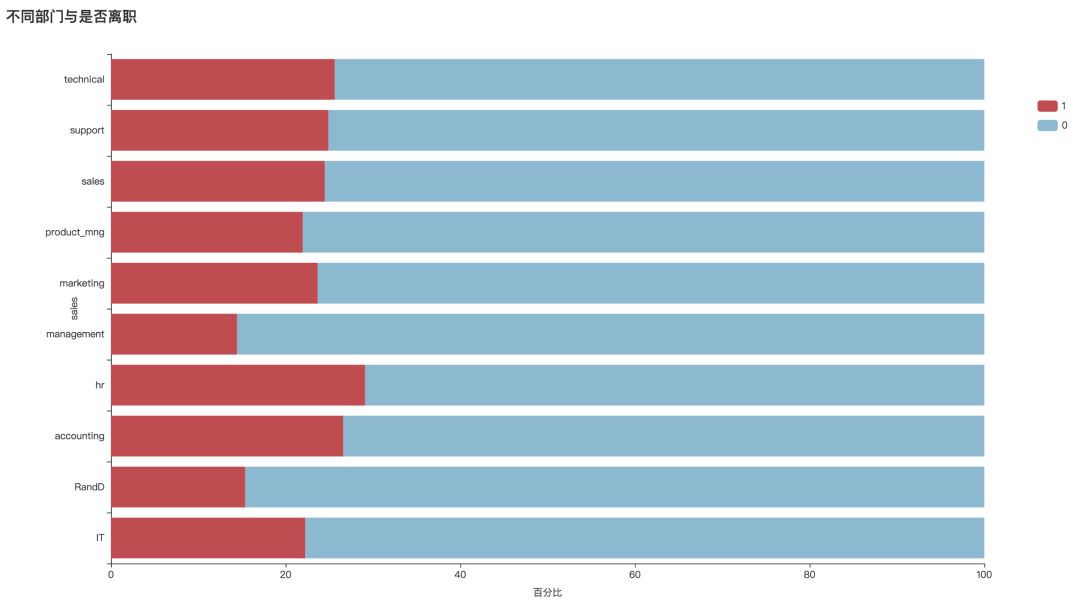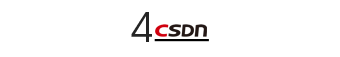• 薪资水平 salary 为定序变量, 因此将其字符型转化为数值型。

• 岗位是定类型变量, 对其进行 one-hot 编码。

``````# 数据转换
df['salary'] = df['salary'].map({"low": 0, "medium": 1, "high": 2})
# 哑变量
df_dummies = pd.get_dummies(df,prefix='sales')
``````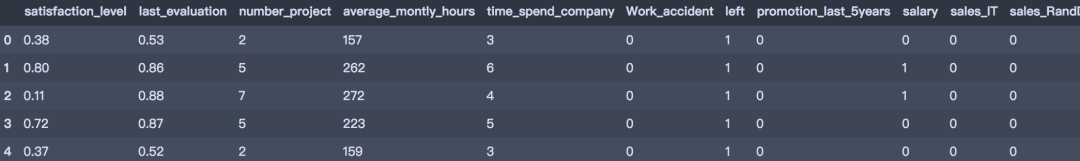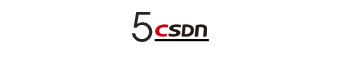``````from sklearn.model_selection import train_test_split, GridSearchCV
from sklearn.tree import DecisionTreeClassifier
from sklearn.ensemble import RandomForestClassifier
from sklearn.metrics import classification_report, f1_score, roc_curve, plot_roc_curve
``````

``````x = df_dummies.drop('left', axis=1)
y = df_dummies['left']

X_train, X_test, y_train, y_test = train_test_split(x, y, test_size=0.2, stratify=y, random_state=2020)
print(X_train.shape, X_test.shape, y_train.shape, y_test.shape)
``````

1、决策树

``````# 训练模型
clf = DecisionTreeClassifier(criterion='gini', max_depth=5, random_state=25)
clf.fit(X_train, y_train)
train_pred = clf.predict(X_train)
test_pred = clf.predict(X_test)

print('训练集：', classification_report(y_train, train_pred))
print('-' * 60)
print('测试集：', classification_report(y_test, test_pred))
``````
``````训练集：               precision    recall  f1-score   support

0       0.98      0.99      0.98      9142
1       0.97      0.93      0.95      2857

accuracy                           0.98     11999
macro avg       0.97      0.96      0.97     11999
weighted avg       0.98      0.98      0.97     11999

------------------------------------------------------------

0       0.98      0.99      0.98      2286
1       0.97      0.93      0.95       714

accuracy                           0.98      3000
macro avg       0.97      0.96      0.97      3000
weighted avg       0.98      0.98      0.98      3000

``````

``````# 重要性
imp = pd.DataFrame([*zip(X_train.columns,clf.feature_importances_)], columns=['vars', 'importance'])
imp.sort_values('importance', ascending=False)
imp = imp[imp.importance!=0]
imp
``````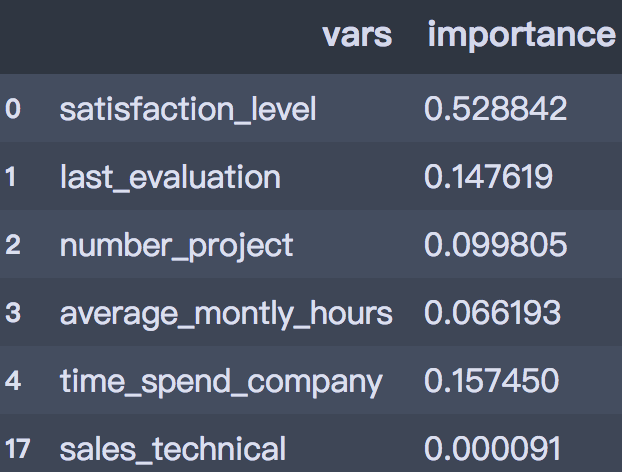``````parameters = {'splitter':('best','random'),
'criterion':("gini","entropy"),
"max_depth":[*range(1, 20)],
}

clf = DecisionTreeClassifier(random_state=25)
GS = GridSearchCV(clf, parameters, cv=10)
GS.fit(X_train, y_train)

print(GS.best_params_)

print(GS.best_score_)
``````
``````{'criterion': 'gini', 'max_depth': 15, 'splitter': 'best'}
0.9800813177648042
``````

``````# 训练模型
clf = DecisionTreeClassifier(criterion='gini', max_depth=5, random_state=25)
clf.fit(X_train, y_train)
train_pred = clf.predict(X_train)
test_pred = clf.predict(X_test)

print('训练集：', classification_report(y_train, train_pred))
print('-' * 60)
print('测试集：', classification_report(y_test, test_pred))
``````
``````训练集：               precision    recall  f1-score   support

0       0.98      0.99      0.98      9142
1       0.97      0.93      0.95      2857

accuracy                           0.98     11999
macro avg       0.97      0.96      0.97     11999
weighted avg       0.98      0.98      0.97     11999

------------------------------------------------------------

0       0.98      0.99      0.98      2286
1       0.97      0.93      0.95       714

accuracy                           0.98      3000
macro avg       0.97      0.96      0.97      3000
weighted avg       0.98      0.98      0.98      3000
``````

2、随机森林

``````rf_model = RandomForestClassifier(n_estimators=1000, oob_score=True, n_jobs=-1,
random_state=0)
parameters = {'max_depth': np.arange(3, 17, 1) }
GS = GridSearchCV(rf_model, param_grid=parameters, cv=10)
GS.fit(X_train, y_train)

print(GS.best_params_)
print(GS.best_score_)
``````
``````{'max_depth': 16}
0.988582151793161
``````
``````train_pred = GS.best_estimator_.predict(X_train)
test_pred = GS.best_estimator_.predict(X_test)

print('训练集：', classification_report(y_train, train_pred))
print('-' * 60)
print('测试集：', classification_report(y_test, test_pred))

``````
``````训练集：               precision    recall  f1-score   support

0       1.00      1.00      1.00      9142
1       1.00      0.99      0.99      2857

accuracy                           1.00     11999
macro avg       1.00      0.99      1.00     11999
weighted avg       1.00      1.00      1.00     11999

------------------------------------------------------------

0       0.99      0.98      0.99      2286
1       0.95      0.97      0.96       714

accuracy                           0.98      3000
macro avg       0.97      0.98      0.97      3000
weighted avg       0.98      0.98      0.98      3000
``````

• 属性：数值型数据常常是模型不稳定的来源，可考虑对其进行分箱；重要属性筛选和字段扩充；

• 算法：其他的集成方法；不同效能评估下的作法调整。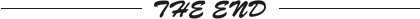``````更多精彩推荐
☞Kubernetes 并非灵丹妙药...
☞“云”话数字经济：2020 腾讯全球数字生态大会定档 9 月！
☞蚂蚁上市分给员工 7000 亿股权，曾有人 28 岁财务自由，这次又将产生多少千万富翁？
☞MIT 更新最大自然灾害图像数据集，囊括 19 种灾害事件
☞闲鱼的云原生故事：靠什么支撑起万亿的交易规模？
☞云交易所已成资金盘、杀猪盘重灾区，曾被寄予厚望，如今罪恶丛生

``````20年前，《新程序员》创刊时，我们的心愿是全面关注程序员成长，中国将拥有新一代世界级的程序员。20年后的今天，我们有了新的使命：助力中国IT技术人成长，成就一亿技术人！